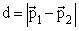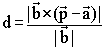Previous  Top  Next

2 pointspoints linepoints plane2 lines:if both not parallel (else point-line)

Line - plane
If the line is parallel to the plane, then the distance is the distance of one point to the plane.

2 planes
If both planes are not parallel, then the distance is the distance of one point of a plane to the plane.